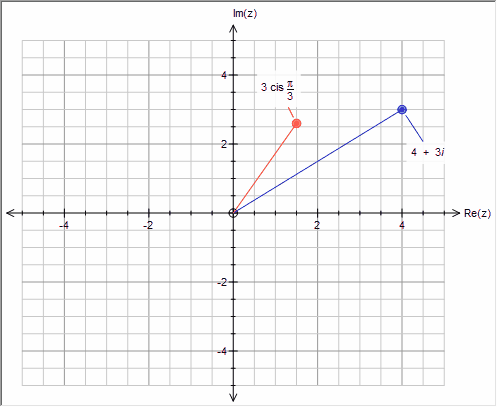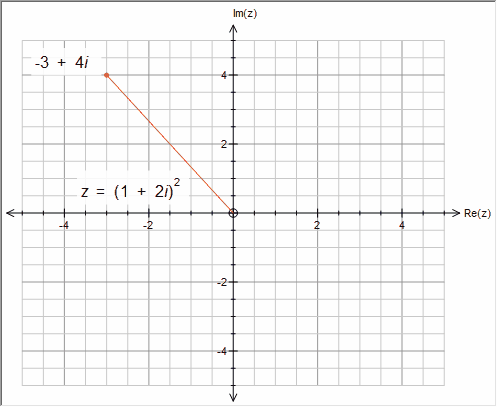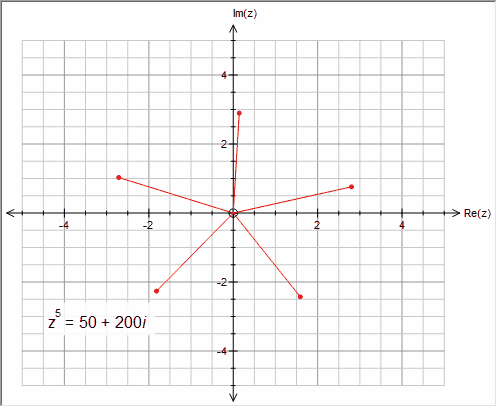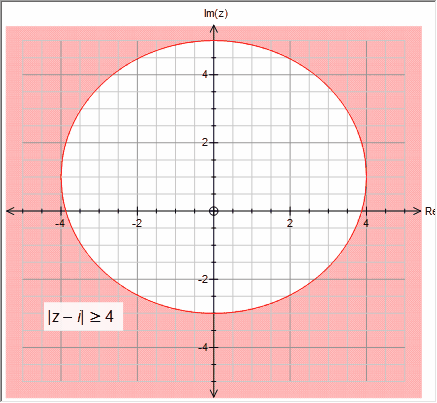﻿ Complex Numbers

# Complex Numbers

Top  Previous  Next
 The graph tool  introduces some support for complex numbers.  It is possible to confuse the graph tool, but in the vast majority of school situations,   it will produce the desired result.   To enter a complex number, just type it in as it is written.   z = 4+3i                z = 3cis(pi/3)Complex calculations will be performed prior to graphing the result   z = (2 + 3i)2The graph tool can also solve some complex equations   z5= 50+200iFinally, the graph tool understands magnitudes and can graph most cases.   | z - i | >= 4